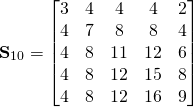# QUARTER RHOMBS

A rhomb may be divided by its diagonals into four right triangles, related by mirror lines along the diagonals. We will call these triangles quarter-rhombs. This designation is chosen to include the mirror images of the right triangular fundamental domain of a rhomb with point symmetry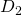. (Note that on the previous page we used the expression half-rhombs for an isosceles triangular fundamental domain of a rhomb with D1 or C2 point symmetry.) On this page we will show that it is possible to construct substitution tiles for right triangles with angles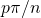,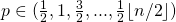, for both odd and even n and inflation factor

(1)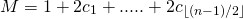where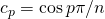.

The lengths of its legs are

(2)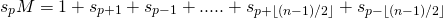(3)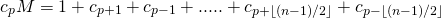All terms of the first equation are positive, but some of the terms in the other two equations are negative. They will eliminate an equal number of positive terms. As a result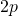terms in the third equation  wil be lost and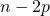terms in the second equation. Because only positive elements are left, the borders of the substitution tile may be constructed by aligning prototile legs having lengths corresponding to these terms along the substitution tile edges. The arrangement order of the prototiles along the borders should be chosen in such a way that it allows a consistent tiling of the inside of the substitution tile. To achieve this, a first strategy is to combine as many pairs of quarter-rhombs as possible into half-rhombs at the borders of the substitution tile. In the figure below the borders of all the right triangular substitution tiles for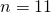are shown as an example.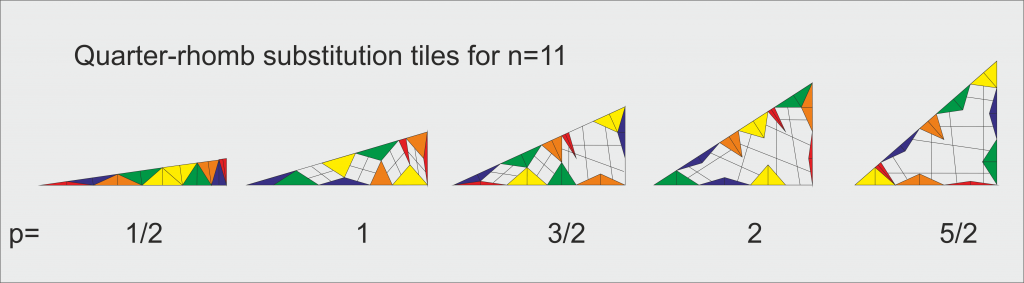Quarter-rhomb tile borders for . Prototile pairs have been aligned along the edges in such a way that the crossings of the lines connecting parallel hypotenuses correspond to positive tiles (see text)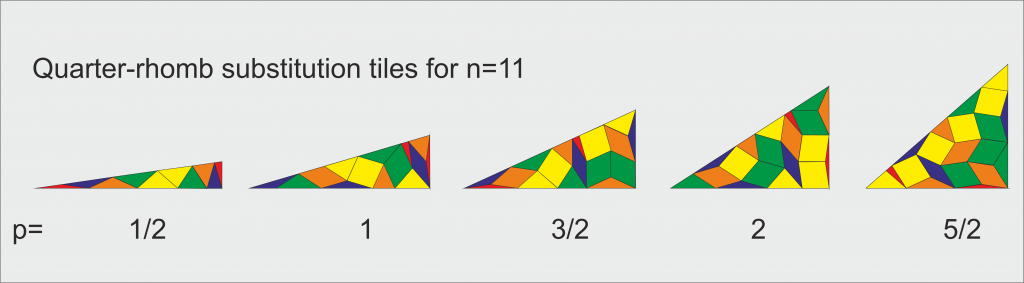Filled in quarter-rhomb substitution tiles for . Quarter-rhombs have been combined into half-rhombs or full-rhombs, wherever possible.

Next, one may try to fill up the interior of the tile with full-rhombs. Because the inflation factor M is the sum of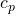terms with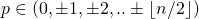, the prototile hypotenuses of the quarter-rhombs along the substitution tile hypotenuse point in n possible directions, between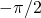and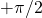with respect to the hypotenuse direction. The same is true for the prototlle hypotenuses along the two legs of the substitution tile. So, there are pairs of parallel prototile hypotenuses, one at the substitution tile hypotenuse and the other one at one of the legs. These pairs may be connected by a finite worm of rhombs. Drawing lines between the pair members one gets crossings. It has been shown by Dirk Frettloeh and Edmund Edwards (Parallelogram tilings, worms and finite orientations, Discrete and Computational Geometry 49 (2013) 531-539) that in a rhomb tiling  two worms are either parallel or cross only once. Each crossing correspondsto a rhomb prototile having edges parallel to the two pairs of worm edges. These rules are very helpful in constructing a valid tiling because all crossings should yield a possitive prototile. If not, the arrangement of tiles along the border may be altered until they do. For the case ofapparently a valid tiling in terms of interior full-rhombs can be found as is illustrated in the above pictures. Half-rhombs appear only at the edges of the supertiles. Because all quarter-rhombs have been combined into half-rhombs in the final tiling, it is in fact a half-rhomb tiling.

The substitution matrix may be found using our general model for rhomb tilings .by subtracting the negative tiles from the corresponding positive ones, adding tiles with the same shape and discarding zero area tiles.

(4)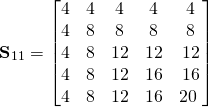Below full-rhomb tiles consisting of four mirror symmetric right triangular prototiles for odd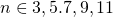are  shown. Note that the smallest prototiles are a set of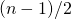quarter-rhombs, not counting the mirror-symmetric prototiles as separate prototiles.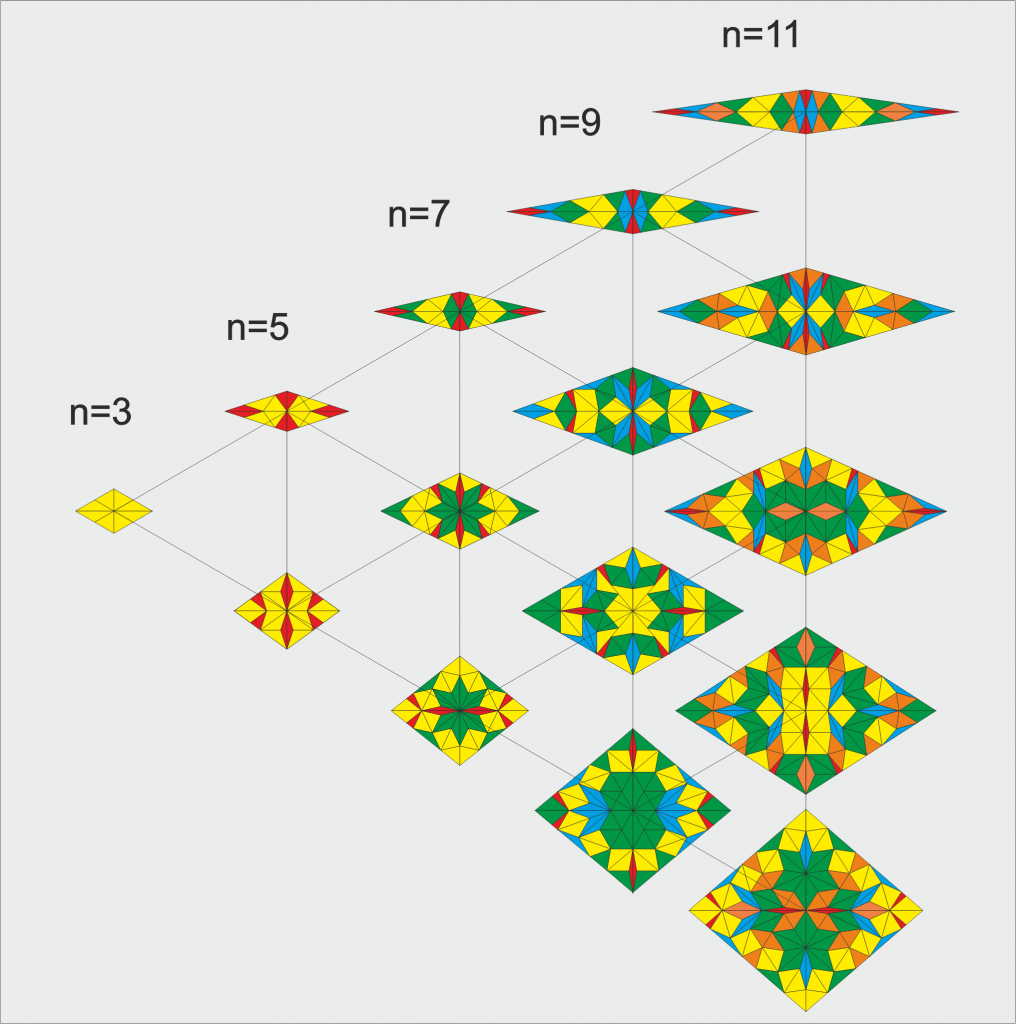Rhomb tiles consisting of 4 mirror symmetric right triangular prototiles for odd n and .

The substitution matrix for odd n and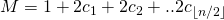is

(5)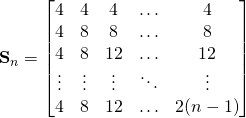This matrix corresponds to four times the matrix on the previous page for half-rhomb tilings for odd n. To see this, one should note that the above tilings may be considered to be half-rhomb tilings as well, but the order of the tiles is different the ones on the previous page, because here we use the opening angle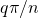of the quarter rhomb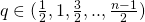, which corresponds to odd-p opening anglesfor isosceles triangles with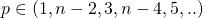.

## Quarter-rhomb Tilings for Even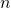.

This special type of aperiodic tiling is not only possible for odd, but also for even. Below the substitution tiles for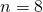are shown. The opening angles are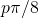with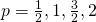.

Because the right triangles always occur in mirrored pairs, the eventual tiling may also be considered to be a half-rhomb tiling with twice as many prototiles.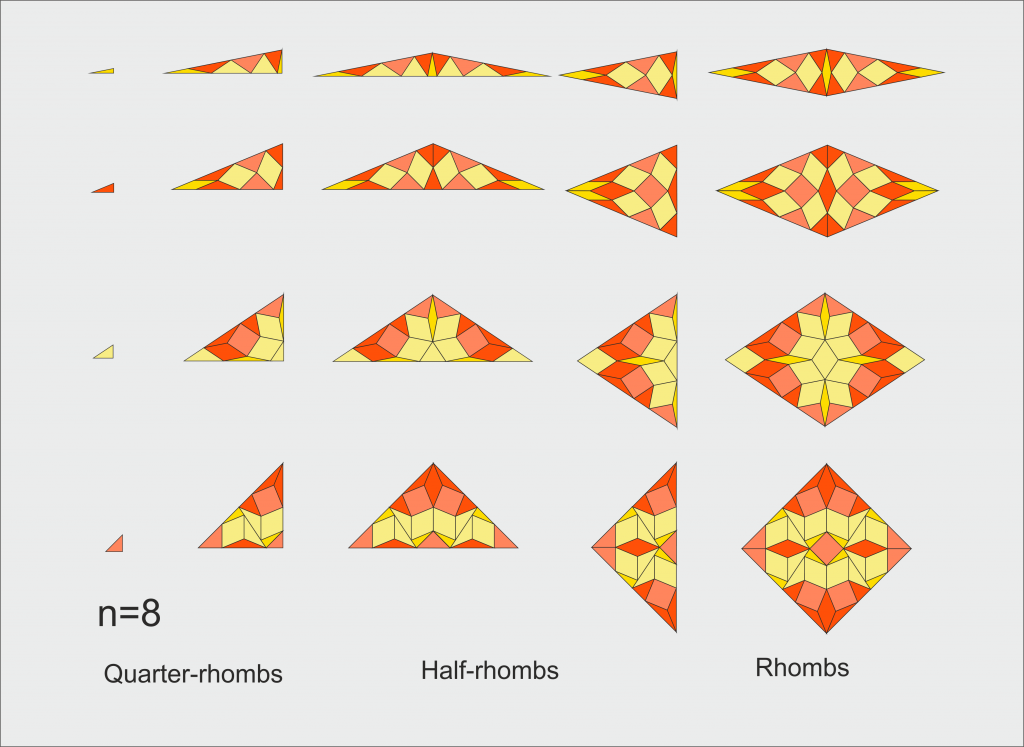Right triangle or Quarter-rhomb tiles for . The opening angles are , , and .

Below a summary of the tiles for even n up to n=10 is shown. The basic prototiles are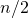quarter-rhomb tiles. Alternatively,half-rhombs may be assigned as prototiles by combining mirror symmetric pairs of quarter-rhombs. In the picture thefull rhombs are shown, which are also important building blocks of the tilings.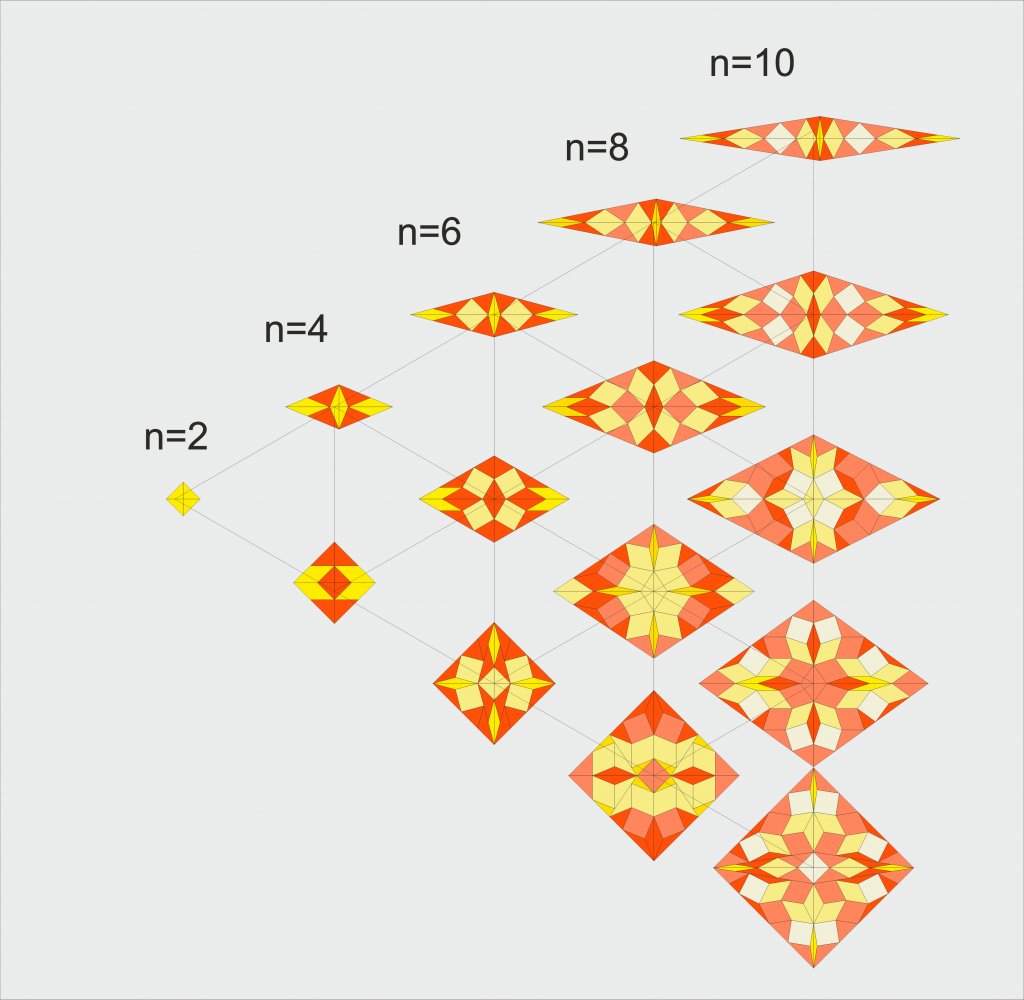Rhomb tiles cosisting of 4 mirror symmetric right triangular prototiles for even and .

The substitution matrix for even n and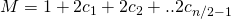is

(6)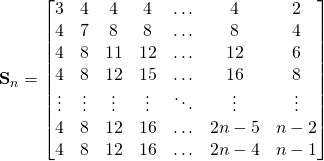i.e.

(7)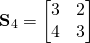(8)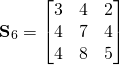(9)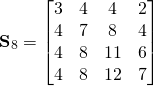(10)